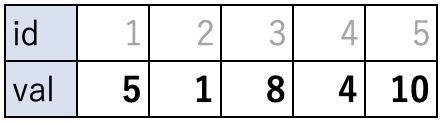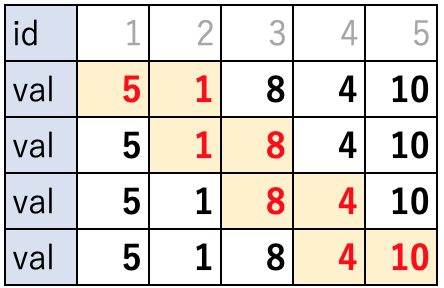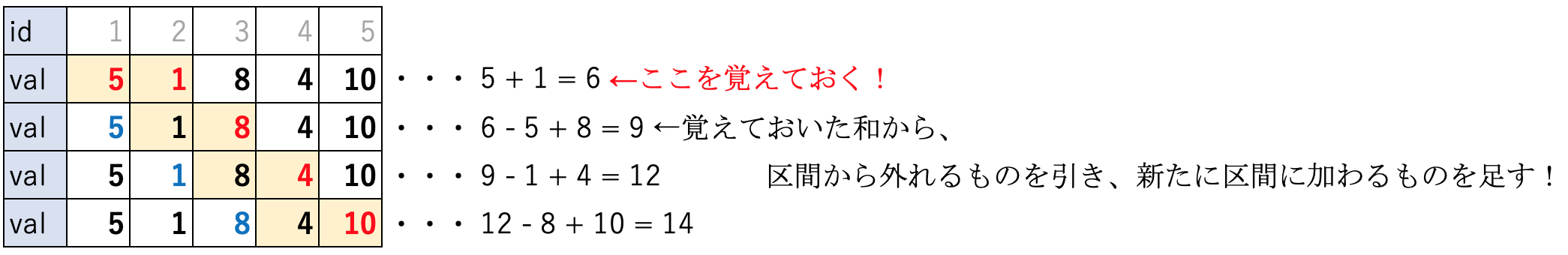# Article summary

This is a series of articles that I have collected in my study and memo. Sixth bullet. Click here for previous articles.

# Articles
5 Introduction to Algorithms in Java-Cumulative Sum
4 Introduction to algorithms in java-Search (bit full search)
3 Introduction to Algorithms in Java-Search (Breadth-first search)
2 Introduction to algorithms in java-Search (depth-first search)
1 Introduction to Algorithms in Java-Search (Full Search, Binary Search)

• Scooping method *

Study about With cumulative sum? Is it the one used? I often see them introduced in the set.

## Scooping method

Like cumulative sums, it seems to be a technique to speed up operations on arrays of numbers.

Well… it’s a bit confusing if you look at the link. .. .. I will explain it for my own study.

As an example, consider an integer array of size n. What is the maximum value of the sum in that interval when it is divided into the specified interval length t? It is a problem that solves a general problem. It is a problem that can be done with cumulative sum. See also previous article.

As an example, consider a size of 5 (n = 5) and an interval length of 2 (t = 2).Consider the array as shown. If you divide this by the length of the interval, it will be as follows.From the conclusion, the sum of 4 + 10 = 14 when choosing the 4th and 5th is the highest value, but how much calculation is so far?

1. Which section to select
2. Calculation of the sum within that section

Let’s divide it into two parts.

First of all, 1. How to divide the section is easy. In the above example, the sum is summed four times. If you drop it into the formula, it becomes n-t + 1 times. This time 5-2 + 1 = 4 times.

Next is 2. Calculation of the sum within that interval, but it is processed twice each to access each element of the array.

In total, (n-t + 1) * t times. If t is dependent on n, the amount of calculation will be about O(n^2), so be careful. In this case, if n is taken as 10^5, it will take a very long time. That’s when the scooping method came into play.

Well, it’s a relatively simple story, but… Please remember the sum and do not have to add from 1 each time. about it. See the figure below.Do you understand it somehow? Remembering the sum, you don’t have to add all the elements t times. The complexity is n-t + 1 times, because you no longer need to access each element of the array. I think it can be expressed as O(n).

Let’s go to the example.

## Example

Example: AtCoder-abc032-c “column”

- -- (Start of section) 【Problem statement】 There is a nonnegative integer sequence S=s1,s2,...,sN of length N and an integer K. Your task is to find the length of the longest continuous subsequence of S that satisfies the following conditions. Substring length must be greater than or equal to 1. - The product of the values of all the elements included in the subsequence is K or less. If there are no substrings that satisfy the condition, output 0. 【input】 Input is given from standard input in the following format: ``` N K s1 s2 : sN ``` ・In the first line, the integer N (1 ≤ N ≤ 105) that represents the length of the sequence and the integer K (0 ≤ K ≤ 10^9) in the question sentence are given separated by spaces. - The values of each element of the numerical sequence are given to the Nth row from the second row. In the i(1≦i≦N) line, si(0≦si≦10^9) is written. 【output】 Output to the standard output in the following format. In the first line, output the length of the longest continuous subsequence whose product of the values of all contained elements is less than or equal to K. If there is no substring that satisfies the condition, output 0. Don't forget the trailing newline. (End of section) - --

This is the problem. It is a little applied. Basically, it’s a scooping method, but this time the length is not fixed. It will be implemented according to the following policy.

• Save the product as you would for the scooping method.
• Proceed to the right end as long as the conditions are met.
• If the condition is no longer met, move to the left end.
• When the left end reaches the far right, the search ends. The longest value at that point is output.

#### `Main.java`

``````
import java.util.Scanner;

public class Main {
public static void main(String[] args) {
Scanner sc = new Scanner(System.in);

int n = sc.nextInt(); // length of non-negative integer sequence
long k = sc.nextLong(); // maximum product

long[] s = new long[n]; // non-negative integer sequence

for (int i = 0; i <n; i++) {
s[i] = sc.nextLong();
if (s[i] == 0l) {
System.out.println(n);
return;
}
}

long seki = 1; // Variable storing the product
int ans = 0; // maximum length
int ans_max = -1; // save answer
int left = 0; // left edge
int right = 0; // right edge

for (left = 0; left <n; left++) {

// Fix the left end, move the right end until you can go to multiplication
while (right <n && seki * s[right] <= k) {
seki *= s[right++];
}

// store the maximum value of ans
ans = right-left;
if (ans_max <ans) {
ans_max = ans;
}

// Move the left end. Be careful when left == right.
if (left == right) {
right++;
} else {
seki /= s[left];
}

}

System.out.println(ans_max);
}
}
``````

Yes. I referred to various sources, but it was difficult. .. It was especially difficult for left to catch up with right. It seems that this also requires practice. .. .. Let’s practice well. I will try to read the source over and over again.

see you!

Tags:

Updated: